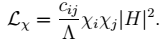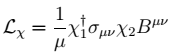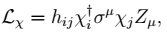# SM + 2 Fermions

It is worth generalizing the previous discussion to the case with two new singlet fermions χ1 and χ2. The Majorana mass matrix for these two fermions has three parameters, and the dimension-five Higgs portal operators form a matrix(1)
After electroweak symmetry breaking, the BSM fermions form two mass eigenstates χ1 and χ2, with mass m2 > m1. If we take relatively light fermions mh > 2 m2, the decays h→ χ2χ2, h→χ1χ2 and h→χ1χ1 are all possible. This kind of interaction appears in, for instance, the NMSSM, where χ2 and χ1 are mostly bino- and singlino-like, respectively, and the higher-dimension Higgs portal coupling of Eq. (1) results after integrating out the charged Higgsinos.
Let us first consider the case where there is a Z2 symmetry which takes χi→ −χi. In this case, χ1 is stable, but the heavier new state decays as χ2 → χ1+X. If the Higgs portal coupling of Eq. (1) is the only coupling of the χi, then the decay will proceed through an off-shell Higgs, χ2h*χ1ff (gg, γγ) χ1. In this case, branching fractions into different SM partons will be determined by the Higgs couplings, and will typically result in Higgs decays to E/T plus one or two non-resonant quark-antiquark, lepton-anti-lepton, or gluon pairs, depending on the available phase space.
If the χi have additional interactions besides their coupling to the Higgs, such as a dipole coupling to the hypercharge field strength,(2)

or a coupling to the Z boson induced by mixing with states transforming under SU(2)L,(3)
then other decay patterns are possible. The dipole operator allows the decays χ2→γχ1, as well as χ2→χ1 Z if m2m1 > mZ (phase space suppression renders decays through an off-shell Z largely irrelevant when m2m1 < mZ). The operator of Eq. (3) also yields χ2→ χ1 Z when phase space allows, or if m2m1 < mZ, will mediate the three-body decays χ2ff χ1 with branching ratios set by the Z branching fractions.
Note that a common feature of all these decays is that the pairs of SM partons have a kinematic endpoint at mff, gg,γγ < m2m1, and that the transverse mass of the visible partons and the E/T is bounded from above.
The Z boson coupling can arise in NMSSM-like models, see e.g. NMSSMS, or in models with additional RH neutrinos [1,2] that mix with the SM neutrinos. In the latter case, the couplings hij in (3) are usually small and therefore the neutrino decay lengths are macroscopic. In the former case, the couplings can instead be larger, and the Majorana fermions can have a prompt decay into SM fermions. Additional examples are models with a fourth generation of fermions where the two fourth generation neutrinos do not mix with the SM neutrinos [3,4,5]. In these models, the mass range M1 >~30 GeV, M2M1 <~20 GeV is allowed by LEP measurements of the Z width and LEP bounds on e+e→ χ1 χ2, χ2 χ2 . In this region of parameter space, h→ χ2 χ2, as well as h→χ1 χ1, can have a sizable branching ratio . Furthermore, the heavier neutrino χ2 can decay promptly via χ2Z* χ1, while the lighter neutrino χ1 is long-lived.
If the Z2 parity is violated, allowing χ1 to decay, Higgs decays to as many as ten partons may result. We will not consider such complex decays in this work, but one should bear in mind that they can occur.
Many models with new fermion species also contain new bosonic degrees of freedom, which, if light, open new possibilities for the decays of the χi. We will see examples of this in NMSSM+F.

## References

M. L. Graesser, Experimental Constraints on Higgs Boson Decays to TeV-scale Right-Handed Neutrinos, [arXiv:0705.2190].
M. L. Graesser, Broadening the Higgs boson with right-handed neutrinos and a higher dimension operator at the electroweak scale, Phys.Rev. D76 (2007) 075006, [arXiv:0704.0438].
L. M. Carpenter, Fourth Generation Lepton Sectors with Stable Majorana Neutrinos: From LEP to LHC, [arXiv:1010.5502].
W.-Y. Keung and P. Schwaller, Long Lived Fourth Generation and the Higgs, JHEP 1106 (2011) 054, [arXiv:1103.3765].
L. M. Carpenter and D. Whiteson, Higgs Decays to Unstable Neutrinos: Collider             Constraints from Inclusive Like-Sign Dilepton Searches, [arXiv:1107.2123].

File translated from TEX by TTH, version 4.03. On 17 Dec 2013, 23:20.